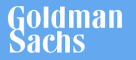New update is available. Click here to update.
Topics

# Valid Arrangement of Pairs

Hard0/120## Problem statement

You are given a 2-d integer array (0-based indexing) “pairs”, where pairs[i] = [ starti, endi ]. Your task is to arrange the given pairs such that for every ‘i’ where 1 <= i <= pairs.length, the endi-1 is equal to starti

Note:
``````The input is generated in such a way that the answer always exists.

If there are multiple answers print any of them.
``````
Example:
``````If pairs = [ [1,2] , [3,1] , [2,3] ] then the following arrangement is valid:

Arrangement =  [ [1,2] , [2,3] , [3,1] ] here, you can see for every ‘i’ where 1 <= i <= 2, the endi-1 is equal to starti
``````
Detailed explanation ( Input/output format, Notes, Images )
Constraints:
``````1 <= T <= 10
1 <= N <= 5 * 10^4
0 <= start , end <= 10^9
starti != endi
No two pairs are exactly the same.

Time limit: 1 sec
``````
Sample Input 1 :
``````2
3
1 2
3 1
2 3
1
1 2
``````
Sample output 1 :
`````` 1 2
2 3
3 1
1 2
``````
Explanation For Sample Output 1:
``````For the first test case, pairs = [ [1,2] , [3,1] , [2,3] ] then the following arrangement is valid:
Arrangement =  [ [1,2] , [2,3] , [3,1] ] here, you can see for every ‘i’ where 1 <= i <= 2, the endi-1 is equal to start

For the second test case, pairs =  [1,2], here the given pair is itself a valid pair.
``````
Sample Input 2 :
``````2
4
1 2
4 5
2 3
3 4
5
1 2
4 5
2 3
3 4
5 1
``````
Sample output 2 :
``````1 2
2 3
3 4
4 5
1 2
2 3
3 4
4 5
5 1
``````Console